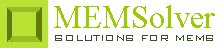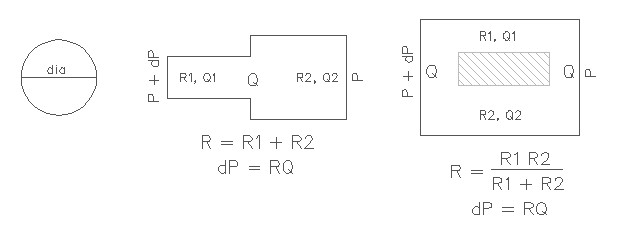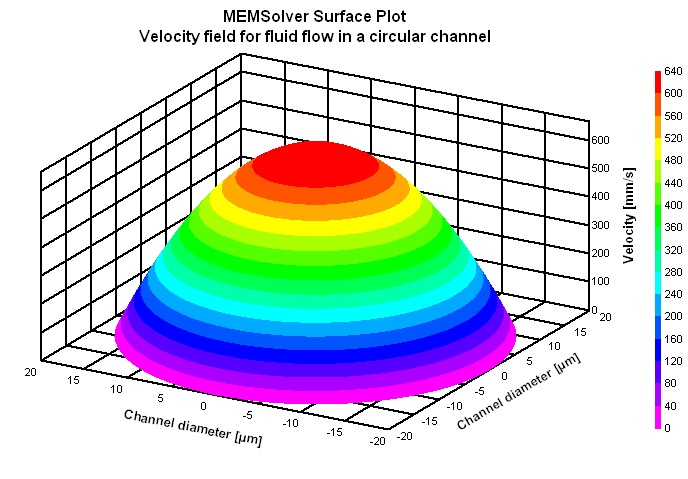CIRCHN,len,dia,dP,vis,sel
Design of a microfluidic channel with circular cross section

len        length of the channel in µm
dia        diameter of the channel in µm
dP         applied pressure difference over the channel length in kPa
vis        dynamic viscosity in mPa-s
sel        number denoting the selected result.
Use 1 for hydraulic flow resistance, 2 for flow rate and 3 for average flow velocityNotes

Pressure driven, steady state flow of an incompressible fluid through a straight microchannel is typically laminar in nature, where the viscous effects dominate and inertial effects can be neglected as characterized by very small Reynolds's number. Viscous effects leads to internal friction in the fluid and creates resistance to the flow of the fluid. Just like electrical resistance leads to Joule heating, hydraulic resistance leads to dissipation of kinetic energy into heat.

Understanding hydraulic resistance is vital to the design of a microchannel. Mathematically it is represented by the Hagen-Poiseuille law that relates constant pressure drop dP resulting in a constant fluid flow Q

dP = Rhyd Q

Hagen-Poiseuille law is analogous to the Ohm's law dV = RI that relates the current I through a wire of resistance R and an electrical potential drop of dV. Similar to electrical resistors connected in series, two channels with different hydraulic resistance can be modeled by summing the two hydraulic resistance together as shown in the picture above. On similar lines, two hydraulic resistors connected in parallel follows the law of additivity of inverse of their hydraulic resistances as shown in the figure.

Use this design form to calculate the hydraulic resistance of a circular microfluidic channel with a constant diameter. If the channel has varying cross sectional area, the hydraulic resistance for individual channels with constant cross section can be found using this design form and then added together using the additivity law explained above. The flow rate can then be estimated using the Hagen-Poiseuille law. The average flow velocity is approximately one half of the maximum velocity which is at the center of the channel. To find the time the fluid takes to travel the full length of the channel, divide the length of the channel with the flow velocity.

The plot shows the velocity field for the Poiseuille flow in a circular channel. It shows the magnitude of velocity at every point on a line along the diameter. The maximum velocity can be found out from the velocity field plot which happens at the center of the channel. The channel diameter cannot be smaller than a minimum value as it will lead to blocking of the channel by air bubbles. That depends on the surface tension of the fluid and the applied pressure.Assumptions

-The Reynold's number is small Re << 1 and inertial effects can be neglected.
-The channels are long, rigid and narrow.
-No-slip boundary condition is assumed.
-The fluid has constant viscosity.
-Perfect hydrostatic pressure balance is assumed in the plane of the channel.